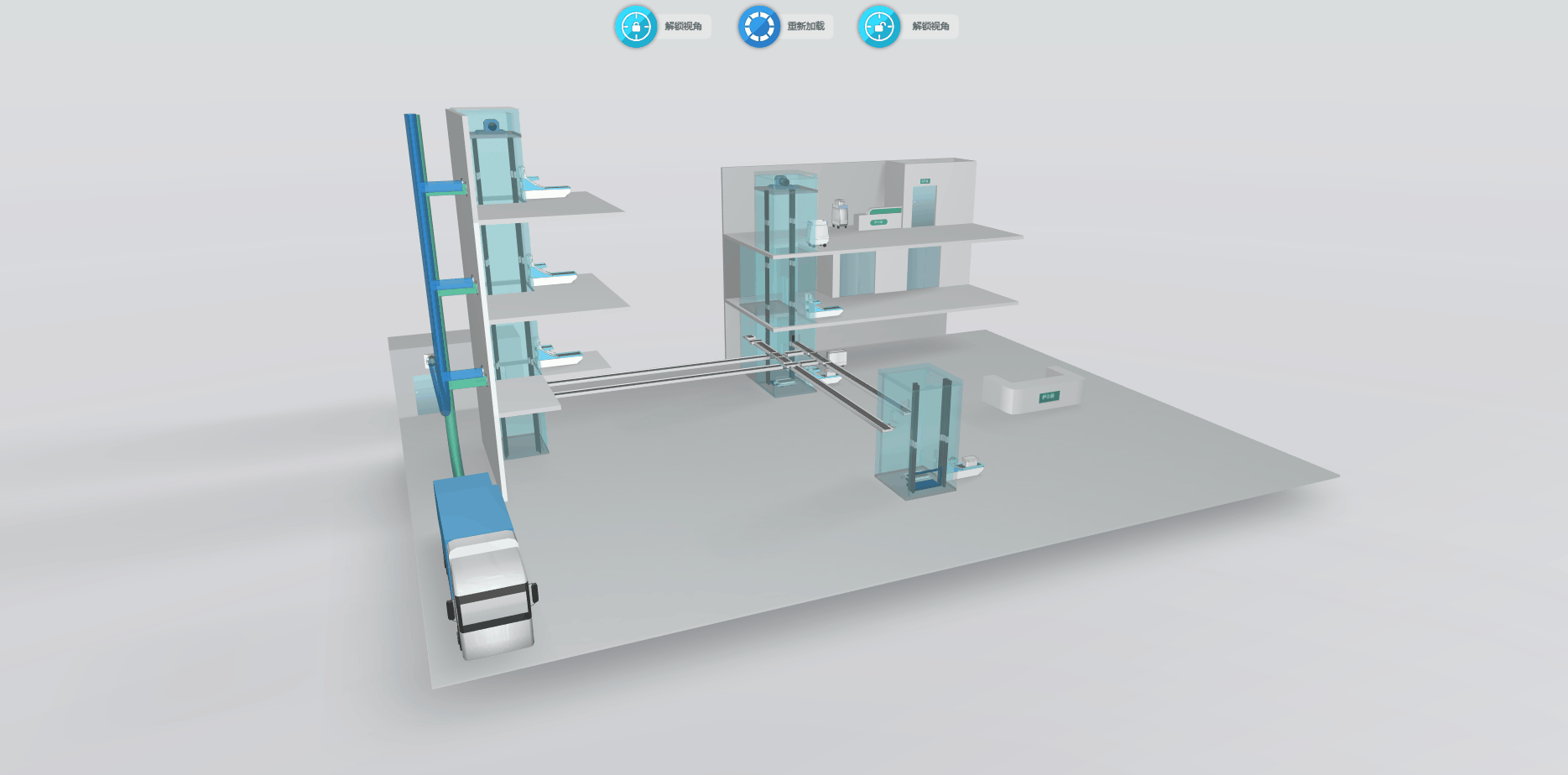2019-10-18 11:29:56

# 代码实现（注：gif 的上传大小有限，实际效果与还请参考 demo 链接）

```gv.setMovableFunc(() => { return false }) // 禁止拖动 gv.getWireframe = (d) => { d.s('wf.visible', false) } // 隐藏选中边框 gv.setEye([583, -212, -789]) // 设置眼睛 gv.setCenter([-76, -654, -133]) // 设置中心点 gv.setFar(100000) // 设置远端位置 gv.setNear(10) // 设置近端位置 gv.setInteractors([ new ht.graph3d.MapInteractor(gv) ]) // 设置交互限制 gv.setSkyBox(dm.getDataByTag('skyBox')) // 设置天空球 window.document.oncontextmenu = () => { return false } // 全局设置右键菜单禁用 gv.scene = { // 复制初始位置  eye: ht.Default.clone(gv.getEye()),
center: ht.Default.clone(gv.getCenter()),
far: ht.Default.clone(gv.getFar()),
near: ht.Default.clone(gv.getNear()),
}```

```gv.mi(e => {
let data = e.data
let kind = e.kind if (kind === 'doubleClickBackground') { // 双击背景 gv.moveCamera(this.gv.scene.eye, this.gv.scene.center, {duration : 1000}) // 恢复视角  } else if (kind === 'doubleClickData') { // 双击模型 gv.flyTo(data, {animation : {duration : 500}, distance : 800}) // 拉近视角  }
})```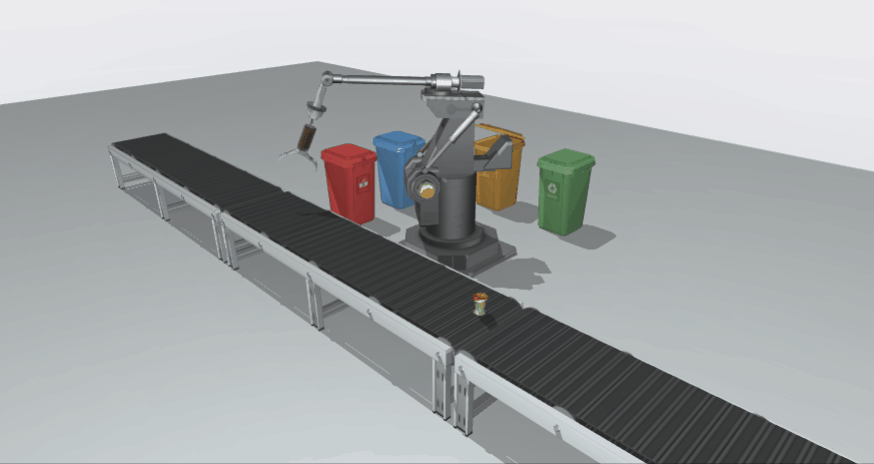```function mechanicalArmAnim1() {
ht.Default.startAnim({
duration: 1000,
easing: (t) => { return t },
action: (v, t) => {
postbrachium.r3(degrees(0) + (degrees(20) - degrees(0)) * v, postbrachium.r3(), postbrachium.r3()) // 后臂向下移 　　　　},
finishFunc: () => {
setTimeout(() => {
mechanicalArmAnim2()
}, 300)
}
})
} function mechanicalArmAnim2() {
ht.Default.startAnim({
duration: 1000,
easing: (t) => { return t },
action: (v, t) => {
postbrachium.p3(-208 + (-184 + 208) * v, postbrachium.p3(), postbrachium.p3()) // 后臂前伸 　　　　　　hydraulicRod1.r3(degrees(0) + (degrees(8) - degrees(0)) * v, hydraulicRod1.r3(), hydraulicRod1.r3()) // 液压杆1倾斜 　　　　　　extensionRod1.r3(degrees(0) + (degrees(8) - degrees(0)) * v, extensionRod1.r3(), extensionRod1.r3()) // 伸长杆1倾斜 　　　　　　extensionRod1.p3(-169 + (-185 + 169) * v, -516 + (-511 + 516) * v, extensionRod1.p3()) // 伸长杆1伸长 　　　　　　hydraulicRod2.r3(degrees(0) + (degrees(-8) - degrees(0)) * v, hydraulicRod2.r3(), hydraulicRod2.r3()) // 液压杆2倾斜 　　　　　　extensionRod2.r3(degrees(0) + (degrees(-8) - degrees(0)) * v, extensionRod2.r3(), extensionRod2.r3()) // 伸长杆2倾斜 　　　　　　extensionRod2.p3(-169 + (-185 + 169) * v, -516 + (-511 + 516) * v, extensionRod2.p3()) // 伸长杆2伸长 　　　　},
finishFunc: () => {
setTimeout(() => {
mechanicalArmAnim3()
}, 300)
}
})
} function mechanicalArmAnim3() {
let oldValue = antebrachium.r3()
ht.Default.startAnim({
duration: 1000,
easing: (t) => { return t },
action: (v, t) => {
hydraulicRod1.r3(degrees(8) + (degrees(7) - degrees(8)) * v, hydraulicRod1.r3(), hydraulicRod1.r3()) // 液压杆1倾斜 　　　　　　extensionRod1.r3(degrees(8) + (degrees(7) - degrees(8)) * v, extensionRod1.r3(), extensionRod1.r3()) // 伸长杆1倾斜 　　　　　　extensionRod1.p3(-185 + (-186 + 185) * v, -511 + (-507 + 511) * v, extensionRod1.p3()) // 伸长杆1伸长 　　　　　　hydraulicRod2.r3(degrees(-8) + (degrees(-7) - degrees(-8)) * v, hydraulicRod2.r3(), hydraulicRod2.r3()) // 液压杆2倾斜 　　　　　　extensionRod2.r3(degrees(-8) + (degrees(-7) - degrees(-8)) * v, extensionRod2.r3(), extensionRod2.r3()) // 伸长杆2倾斜 　　　　　　extensionRod2.p3(-185 + (-186 + 185) * v, -511 + (-507 + 511) * v, extensionRod2.p3()) // 伸长杆2伸长 　　　　　　postbrachium.r3(degrees(20) + (degrees(25) - degrees(20)) * v, postbrachium.r3(), postbrachium.r3()) // 后臂向下移 　　　　　　antebrachium.r3(oldValue + (degrees(-40) - oldValue) * v, antebrachium.r3(), antebrachium.r3()) // 前臂向下移 　　　　　　claw1.r3(degrees(-20) + (degrees(-60) - degrees(-20)) * v, claw1.r3(), claw1.r3()) // 上爪抓取 　　　　　　claw2.r3(degrees(-60) + (degrees(-30) - degrees(-60)) * v, claw2.r3(), claw2.r3()) // 下爪抓取 　　　　},
finishFunc: () => {
mechanicalArmAnim4()
}
})
}```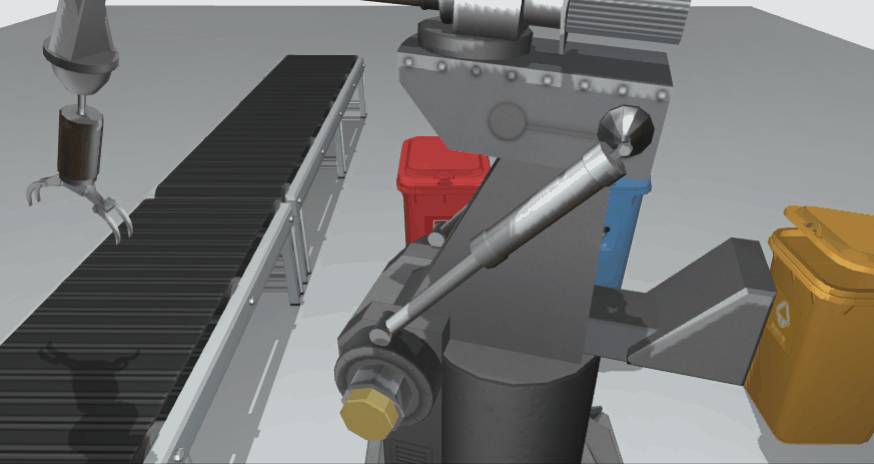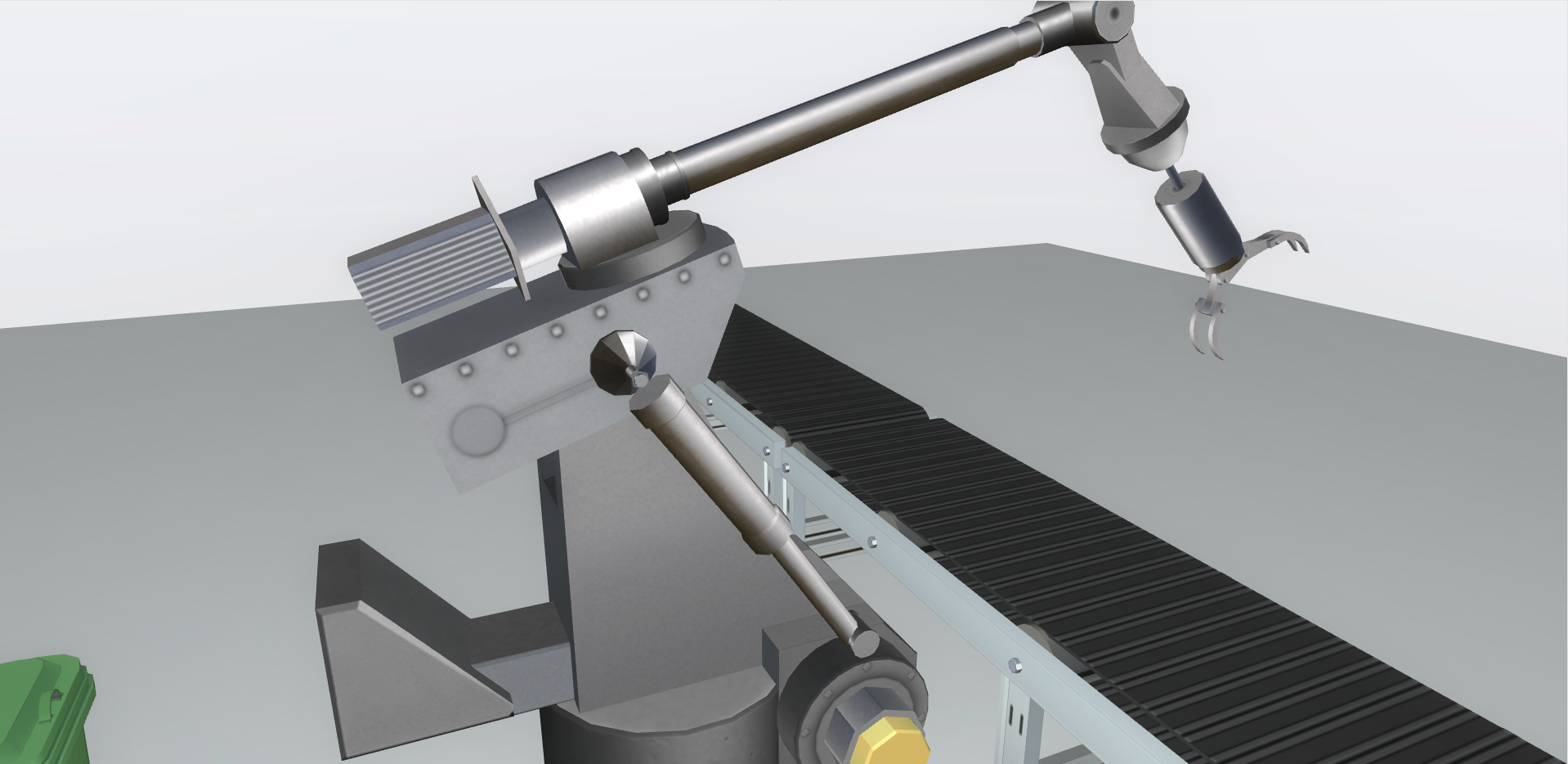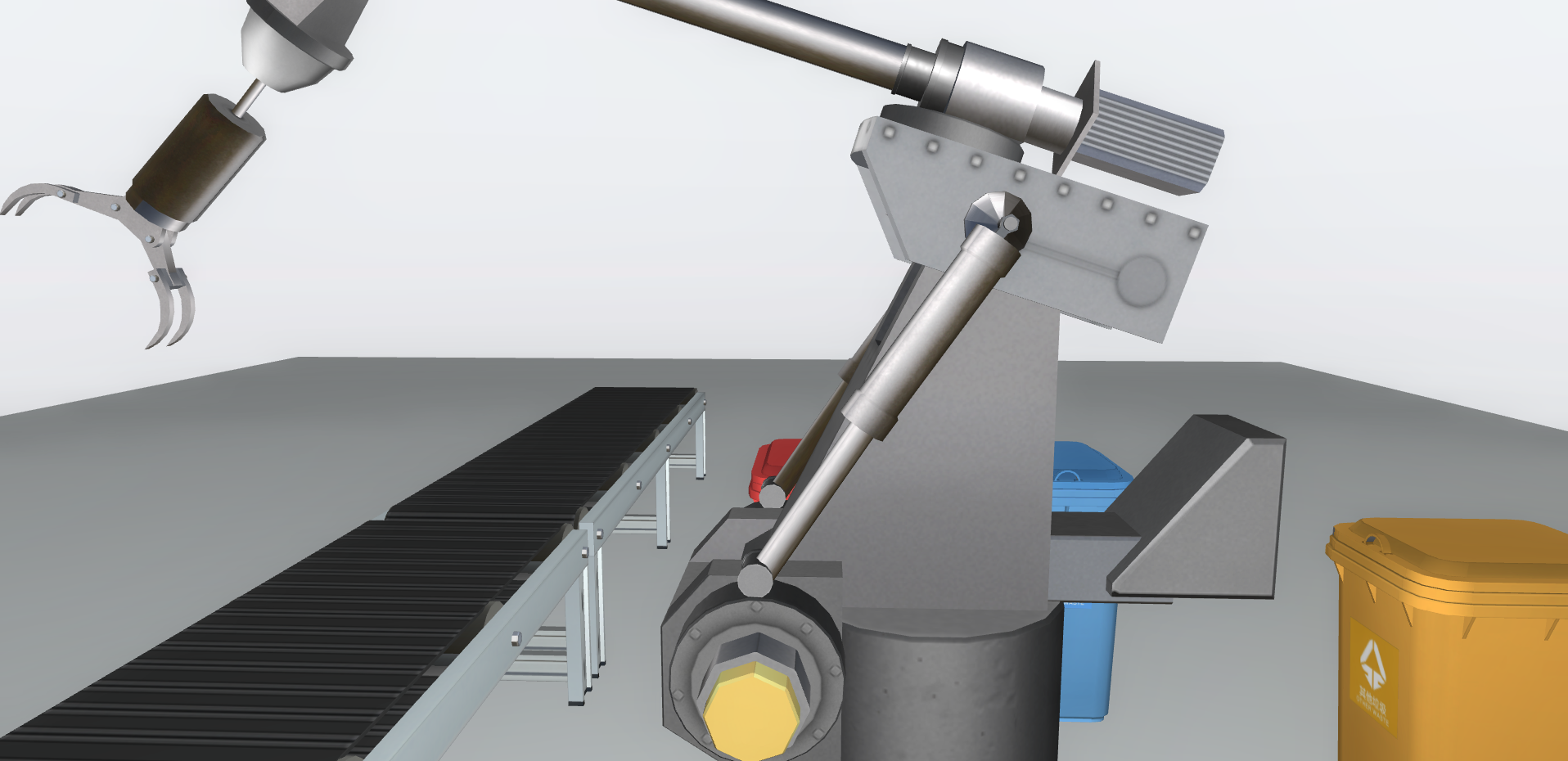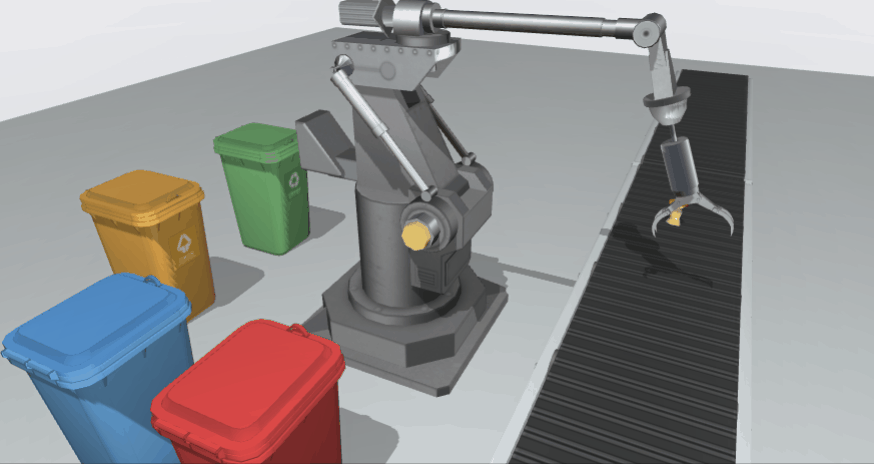```gv.enableShadow(true, {
degreeX : 0, // 投影 x 轴角度 　　degreeZ : -25, // 投影 z 轴角度 　　intensity : 0.3, // 阴影强度， 1 为黑色 　　quality : 'high', // low / medium / high / ultra / 4096数值, 质量 　　type : 'soft', // none / hard / soft 　　radius : 0.2, // type 为 hard / soft 时，补充的边缘厚度，用来提供更柔和的边缘 　　bias : -0.003 // 深度浮点偏差补足 })```

# 总结